Science, Maths & Technology

### Become an OU student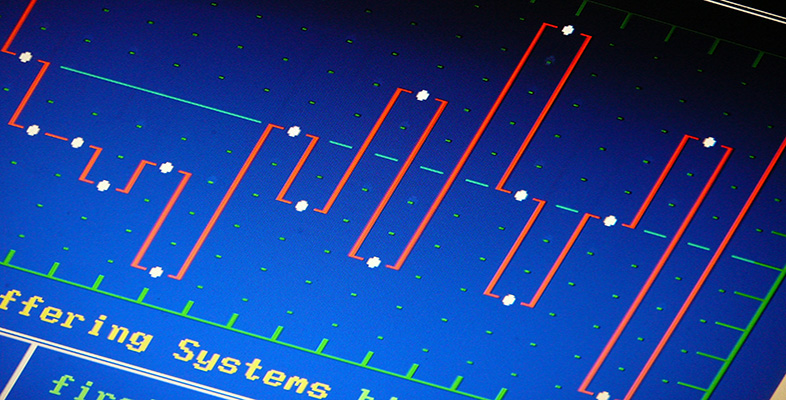Interpreting data: Boxplots and tables

Start this free course now. Just create an account and sign in. Enrol and complete the course for a free statement of participation or digital badge if available.

# 1.2 Boxplot activity

## Activity 1 Drawing a boxplot: chondrite meteors

Table 1.1 contains data on the percentage of silica found in 22 chondrite meteors. The data are given in order of increasing size.

 20.77 22.56 22.71 22.69 26.39 27.08 27.32 27.33 27.57 27.81 28.69 29.36 30.25 31.89 32.88 33.23 33.28 33.4 33.52 33.83 33.95 34.82

(Source: Good, I.J. and Gaskins, R.A. (1980) Density estimation and bump-hunting by the penalized likelihood method exemplified by scattering and meteorite data. J. American Statistical Association, 75, 42-56.)

The median for this data set is 29.025; the lower and upper quartiles are approximately 26.91 and 33.31. The interquartile range is 6.40.

• (a) Using a pencil and ruler, construct a boxplot for these data.

• (b) The sample skewness for these data is −0.446. Is this value in accord with the shape of the boxplot?

### Solution

(a) The data run from 20.77 to 34.82. A convenient scale to cover this range of values runs from 20 to 40. In this case,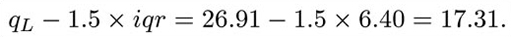This is smaller than the sample minimum, so the left-hand whisker will extend as far as the minimum observation 20.77. (In other words, the lower adjacent value is equal to the sample minimum.) Similarly,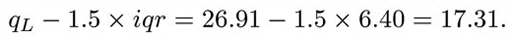This is greater than the sample maximum, so the upper adjacent value is the same as the sample maximum. So with this data set, there are no extreme values to be plotted separately. The boxplot is shown in Figure 3.1.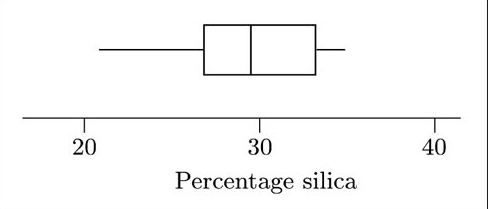Figure 1.5a Boxplot for silica content of chondrite meteors

(b) The sample skewness is negative, indicating that the data are left-skew. To some extent the boxplot reflects this: the left whisker is considerably longer than the right, indicating that the smaller values are more spread out than are the larger values. However, the box gives a different impression. The box corresponds to the middle half of the data values, and the line denoting the median divides this into two parts, each corresponding to one-quarter of the data. In this case, the left part of the box is shorter than the right part. In other words, the box suggests that the data might be right-skew rather than left-skew. So the pattern of asymmetry of these data is not straightforward.

In assessing patterns of skewness from a boxplot, you are looking at five different values: the upper and lower adjacent values, the upper and lower quartiles, and the median. It is thus possible, in some cases at least, to observe somewhat complicated patterns of skewness. On the other hand, calculating the sample skewness involves boiling the data down to a single value; and thus the sample skewness provides rather less information than a boxplot does about the shape of a data set.

The boxplot for the data in Table 1.1, which you were asked to draw in Activity 1, is shown in Figure 1.5.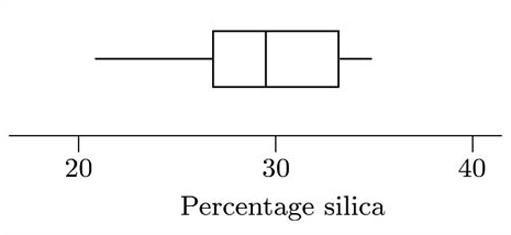Figure 1.5b A boxplot for silica content of chondrite meteors

This boxplot is clearly not symmetrical. However, the pattern of its skewness is not straightforward. The box, corresponding to the middle 50% of the data, appears to be right-skew, because the line marking the median is towards the left of the box (so that the right section of the box is longer than the left). However, the longer whisker is on the left, indicating a longer tail towards smaller values, which in turn suggests that the data are left-skew.

In this example, the sample skewness (−0.446) is in accord with the pattern suggested by the whiskers of the boxplot (left-skew), rather than with that suggested by the box. Essentially, this occurs because all the values in the data set are used to calculate the sample skewness; and the calculation involves a sum of powers of values, so that the sample skewness is particularly affected by the more extreme values in the data set. In a boxplot, the whiskers correspond to the more extreme values. In Figure 1.5, the whiskers suggest that the data are left-skew, matching the sample skewness.